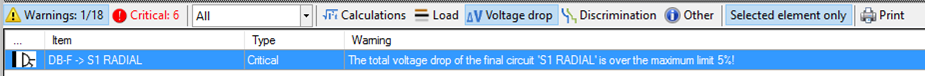Voltage drop tab contains variables and settings about voltage drop calculations for the selected circuit. It has to be reminded that the voltage drop limit value is set at Edit Source window, see Source. The contents of the Voltage Drop tab varies depending on the circuit being a distribution circuit of a final circuit.If the selected circuit is a distribution circuit, then, the user can opt to split the voltage drop limit between the distribution circuit and the final circuit or to take the voltage drop limit as a single value for the whole circuit including the distribution circuit and the final circuit.

If the user decides to split the limit between distribution and the final circuit then a percentage must be set to reserve for the distribution circuit.This is done by typing in a percentage to the text box below Voltage drop limit for the distribution circuit (%) to reserve for the distribution circuit and leave the rest to the final circuit. ElectricalOM will also display the corresponding voltage to the percentage set by the user next to the percentage box. If a distribution circuit is fed from another distribution circuit and both are set to split the voltage drop limit, then, ElectricalOM will let the voltage drop to go as low as the set value for the distribution circuits individually and leave the remaining part for the final circuits connected to the related distribution circuit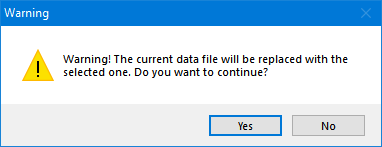The final circuit part depends on the Source settings, for example, if the source is a public supply, then, the total voltage drop for a lighting circuit will be set as 3%. ElectricalOM will display remaining part under Voltage drop limit within the connected final circuits (%). If the user has already set a value manually for the voltage drop, than ElectricalOM will display this value as the total voltage drop limit percentage but otherwise it will assume a total of 5% voltage drop limit at the voltage drop tab for a distribution circuit.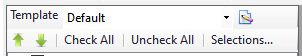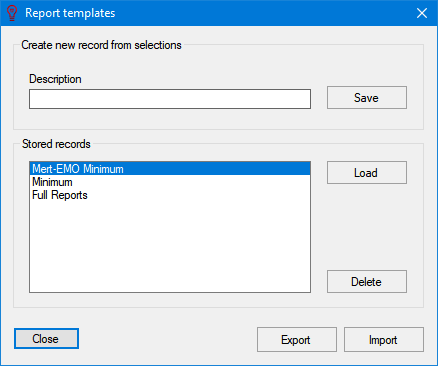ElectricalOM will also take into consideration of the length of the feeding conductor of the selected circuit. If the length is greater than 100 metres, then ElectricalOM will allow an extra 0.005% per metre, and this will be shown as "Extra allowance" under Total voltage drop limit (%) section.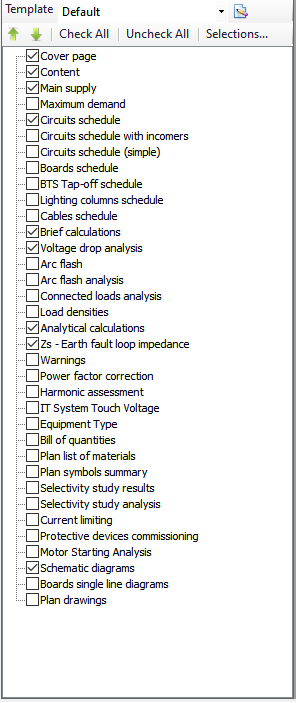If the selected circuit is a final circuit, then, the user can opt to use the remaining part of the voltage drop limit from the distribution circuit or to set a new total voltage drop percentage for the selected circuit manually. If the user sets a manual limit using the User defined option, then there will be two consequences:

• If the distribution circuit is set to Without the split of the voltage drop limit, then, ElectricalOM will define the total voltage drop limit to be the manually set value for the selected circuit including the distribution circuit and the final circuit parts.
• If the distribution circuit is set to Split the voltage drop limit between the distribution circuit and to its connected final circuits, then, ElectricalOM will still reserve the Voltage drop limit of the distribution circuit (%) value for the distribution circuit and leave the rest of the manually set limit for the selected final circuit.

The lower part of the Voltage Drop tab is reserved for the Total Voltage Drop data for the circuit selected. ElectricalOM will display the total voltage drop on the selected circuit on percentage and if there is an issue a red mark will be displayed. Red mark will be placed both on the Voltage Drop tab and next to the phase(s) which has the issue.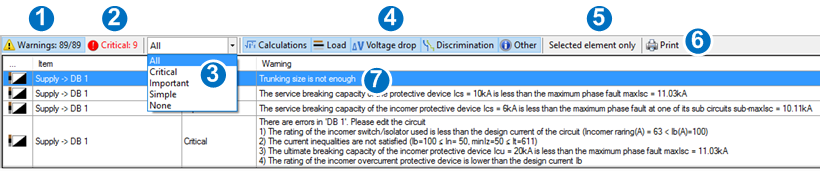It should be noted that Total Voltage Drop section is for the voltage drop at the end of the selected circuit, distribution or final, inducing the distribution circuit which the selected circuit s connected. It also important to note that a distribution circuit can be fed from another distribution circuit, so a final circuit's total voltage drop may include multiple distribution circuits. If the user wants to see the voltage drop associated only with the selected circuit from its origin and end point, then, left hand side of the Circuit Edit Module shall be checked where the calculations and checks are located.